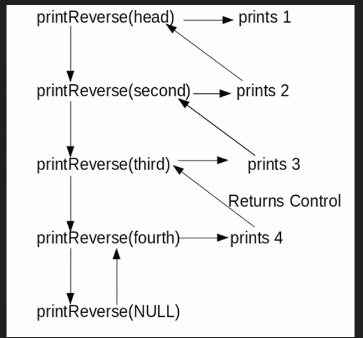# C++ Program For Printing Reverse Of A Linked List Without Actually Reversing

• Last Updated : 24 Dec, 2021

Given a linked list, print reverse of it using a recursive function. For example, if the given linked list is 1->2->3->4, then output should be 4->3->2->1.
Note that the question is only about printing the reverse. To reverse the list itself see this
Difficulty Level: RookieAlgorithm:

```printReverse(head)
1. call print reverse for head->next

Implementation:

## C++

 `// C++ program to print reverse of a linked list``#include ``using` `namespace` `std;` `// Link list node``class` `Node``{``    ``public``:``    ``int` `data;``    ``Node* next;``};` `// Function to reverse the``// linked list``void` `printReverse(Node* head)``{``    ``// Base case``    ``if` `(head == NULL)``    ``return``;` `    ``// Print the list after head node``    ``printReverse(head->next);` `    ``// After everything else is printed,``    ``// print head``    ``cout << head->data << ``" "``;``}` `// UTILITY FUNCTIONS``/* Push a node to linked list.``   ``Note that this function``   ``changes the head */``void` `push(Node** head_ref,``          ``char` `new_data)``{``    ``// Allocate node``    ``Node* new_node = ``new` `Node();` `    ``// Put in the data``    ``new_node->data = new_data;` `    ``// Link the old list off the``    ``// new node``    ``new_node->next = (*head_ref);` `    ``// Move the head to point to``    ``// the new node``    ``(*head_ref) = new_node;``}` `// Driver code``int` `main()``{``    ``// Let us create linked list``    ``// 1->2->3->4``    ``Node* head = NULL;``    ``push(&head, 4);``    ``push(&head, 3);``    ``push(&head, 2);``    ``push(&head, 1);``    ` `    ``printReverse(head);``    ``return` `0;``}``// This code is contributed by rathbhupendra`

Output:

`4 3 2 1`

Time Complexity: O(n)

Please refer complete article on Print reverse of a Linked List without actually reversing for more details!

My Personal Notes arrow_drop_up